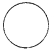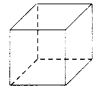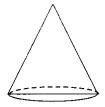# CBSEtips.in

## Wednesday 24 February 2021

### CBSE Class 8 Maths - MCQ and Online Tests - Unit 10 - Visualising Solid Shapes

#### CBSE Class 8 Maths – MCQ and Online Tests –  Unit 10 – Visualising Solid Shapes

Every year CBSE students attend Annual Assessment exams for 6,7,8,9,11th standards. These exams are very competitive to all the students. So our website provides online tests for all the 6,7,8,9,11th standards’ subjects. These tests are also very effective and useful for those who preparing for any competitive exams like Olympiad etc. It can boost their preparation level and confidence level by attempting these chapter wise online tests.

These online tests are based on latest CBSE syllabus. While attempting these, our students can identify their weak lessons and continuously practice those lessons for attaining high marks. It also helps to revise the NCERT textbooks thoroughly

#### CBSE Class 8 Maths – MCQ and Online Tests – Unit 10 – Visualising Solid Shapes

Question 1.
How many faces does a triangular pyramid have ?
(a) 1
(b) 2
(c) 3
(d) 4.

Question 2.
The base of a prism is:
(a) Circle
(b) Triangle
(c) Square
(d) Any shape

Explanation:
A pyramid is a polyhedron whose base is a polygon (of any number of sides) and whose lateral faces are triangles with a common vertex.

Question 3.
How many vertices does a triangular pyramid have ?
(a) 1
(b) 2
(c) 3
(d) 4.

Question 4.
How many edges does a triangular pyramid have ?
(a) 2
(b) 4
(c) 6
(d) 8.

Question 5.
The name of the shape(a) triangle
(b) cone
(c) cylinder
(d) sphere

Question 6.
The name of the shape is(a) cone
(b) circle
(c) cylinder
(d) cube.

Question 7.
The name of the shape is(a) cuboid
(b) cube
(c) square
(d) cylinder.

Question 8.
The name of the shape is(a) cube
(b) cuboid
(c) sphere
(d) square.

Question 9.
The name of the shape is(a) circle
(b) sphere
(c) cylinder
(d) cone.

Question 10.
A cuboid has how many faces ?
(a) 2
(b) 4
(c) 6
(d) 3.

Question 11.
How many faces does a cube have ?
(a) 6
(b) 4
(c) 3
(d) 2.

Question 12.
How many vertices does a cuboid have ?
(a) 4
(b) 6
(c) 8
(d) 3.

Question 13.
How many edges does a cube have ?
(a) 12
(b) 6
(c) 4
(d) 8.

Question 14.
The name of the shape is(a) cylinder
(b) square
(c) circle
(d) triangle.

Question 15.
The name of the shape is(a) sphere
(b) cylinder
(c) cone
(d) triangle.

Question 16.
The name of the shape is(a) cone
(b) sphere
(c) cuboid
(d) cylinder.

Question 17.
If a polyhedron has 6 vertices and 12 edges. What is the number of faces it has ?
(a) 6
(b) 8
(c) 12
(d) 18

Explanation:
By Euler’s formula we know that:
For any polyhedron,
F + V – E = 2
F = 2 – V + E
F = 2 – 6 + 12 = 8

Question 18.
Number of vertices a cone has:
(a) One
(b) Two
(c) Three
(d) Four

Question 19.
An example of cone is:
(a) Tube
(b) A tent
(c) A ball
(d) A box

Question 20.
How many faces does a triangular prism have ?
(a) 5
(b) 4
(c) 3
(d) 6.

Question 21.
How many vertices does a triangular prism have ?
(a) 6
(b) 5
(c) 4
(d) 3.

Question 22.
How many edges does a triangular prism have ?
(a) 11
(b) 9
(c) 6
(d) 3.

Question 23.
How many faces does a pyramid with square base have ?
(a) 5
(b) 3
(c) 2
(d) 6.

Question 24.
How many vertices does a pyramid with square base have ?
(a) 5
(b)4
(c) 3
(d) 6.

Question 25.
How many edges does a pyramid with square base have ?
(a) 8
(b) 6
(c) 4
(d) 5.

See the following solid and answer the following questions:Question 26.
The number of faces is
(a) 6
(b) 7
(c) 8
(d) 9.

Question 27.
The number of vertices is
(a) 5
(b) 7
(c) 8
(d) 10

Question 28.
The number of edges is
(a) 5
(b) 10
(c) 20
(d) 15.

Question 29.
For a polyhedron, if ‘F’ stands for number of faces, V stands for number of vertices and E stands for number of edges, then which of the following relationships is named as Euler’s formula ?
(a) F + V = E + 2
(b) F + E = V + 2
(c) V + E = F + 2
(d) F+ V = E – 2.

Answer: (a) F + V = E + 2

Question 30.
A cube has ______ faces.
(a) 4
(b) 5
(c) 6
(d) 8

Explanation:
A cube is a three dimensional shape having six faces.

Question 31.
A sphere has how many faces ?
(a) One
(b) Two
(c) Four
(d) None

Explanation:
A sphere is a round-shaped object having no faces and edges.

Question 32.
The top-view of a cone looks like:
(a) A Circle
(b) A Square
(c) A Rectangle
(d) A Triangle

Explanation:
The base of a right-cone is always circular. When we see it from the top, it looks like a circle with its vertex as the center of the circle.

Question 33.
The top-view of a cube looks like a:
(a) Circle
(b) Square
(c) Rectangle
(d) Triangle

Explanation:
Since all the faces of a cube are of equal length.

Question 34.
How many vertices does a cube have ?
(a) 8
(b) 6
(c) 4
(d) 2.

Question 35.
How many edges does a cuboid have ?
(a) 12
(b) 8
(c) 6
(d) 4.